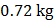# An object of massis tied to a string of lengthand a variable horizontal force is applied on it which starts at zero and gradually increases until the string makes an angelwith the vertical. Work done by the forceisa)b)c)d)## Question ID - 50950 :- An object of massis tied to a string of lengthand a variable horizontal force is applied on it which starts at zero and gradually increases until the string makes an angelwith the vertical. Work done by the forceisa)b)c)d)3537

(c)or(Since, change in kinetic energy is zero)Here,work done by tension = 0work done by fore of gravityNext Question :

A light inextensible string that goes over a smooth fixed pulley as shown in the figure connects two blocks of massesand. Taking, find the work done (in joules) by the string on the block of massduring the first second after the system is released from resta)b)c)d)